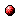# Continuous-state reinforcement learning with fuzzy approximation

Reference:
L. Busoniu, D. Ernst, B. De Schutter, and R. Babuska, "Continuous-state reinforcement learning with fuzzy approximation," Proceedings of the 7th Annual Symposium on Adaptive and Learning Agents and Multi-Agent Systems (ALAMAS 2007) (K. Tuyls, S. de Jong, M. Ponsen, and K. Verbeeck, eds.), Maastricht, The Netherlands, pp. 21-35, Apr. 2007.

Abstract:
Reinforcement learning (RL) is a widely used learning paradigm for adaptive agents. Well-understood RL algorithms with good convergence and consistency properties exist. In their original form, these algorithms require that the environment states and agent actions take values in a relatively small discrete set. Fuzzy representations for approximate, model-free RL have been proposed in the literature for the more difficult case where the state-action space is continuous. In this work, we propose a fuzzy approximation structure similar to those previously used for Q-learning, but we combine it with the model-based Q-value iteration algorithm. We show that the resulting algorithm converges. We also give a modified, serial variant of the algorithm that converges at least as fast as the original version. An illustrative simulation example is provided.Corresponding technical report: pdf file (1.10 MB)
Note: More information on the pdf file format mentioned above can be found here.

Bibtex entry:

@inproceedings{BusErn:07-008,
author={L. Bu{\c{s}}oniu and D. Ernst and B. {D}e Schutter and R. Babu{\v{s}}ka},
title={Continuous-state reinforcement learning with fuzzy approximation},
booktitle={Proceedings of the 7th Annual Symposium on Adaptive and Learning Agents and Multi-Agent Systems (ALAMAS 2007)},
editor={K. Tuyls and S. {de Jong} and M. Ponsen and K. Verbeeck},